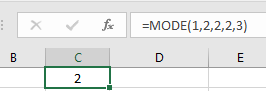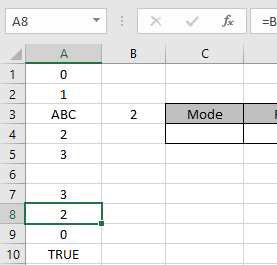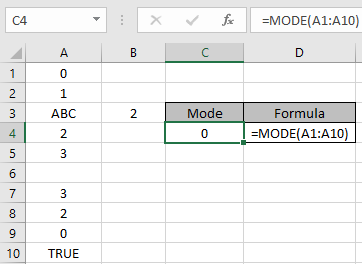# How to use the Excel MODE Function

In this article, we will learn about how to use the MODE function in Excel.

Excel MODE Function is a statistical function of Excel. MODE Function is used to find the most occurring number in the set of array.
Syntax:

=MODE (number1, number2)

Note: It returns a #NA (not applicable) error if no number occurs twice.

For example : =MODE(1,2,2,2,3) // Returns 2
As 2 is most occuring of all of them in array.Let’s understand vividly how to use MODE function in Excel.Here we have random array having numbers, empty cells, boolean values, text & cell references.

Use the Formula in C4 cell

=MODE(A1:A10)As you can see the mode function returns the most occurring number in the array.
You must be wondering why 0 is the most occurring number as 2 & 3 are also occurring the same number of time. But Excel Mode function returns one number only. So it decides between all three numbers and returns the number whichever occur in the array first.

Notes:

• MODE function ignores Text, boolean values & emplty cells.
• MODE function consider numbers, arrays & cell references

Hope you understood how to use MODE function in Excel. Explore more articles on Excel Statistical functions here. Please state your queries in the comment box below. We will help you.

Related Articles:

How to Calculate Mean in Excel

Excel MEDIAN function

Create Histograms in Excel 2016/2013/2010

Regressions in excel

Popular Articles:

50 Excel Shortcuts to Increase Your Productivity

How to use the VLOOKUP Function in Excel

How to use the COUNTIF function in Excel 2016

Terms and Conditions of use

The applications/code on this site are distributed as is and without warranties or liability. In no event shall the owner of the copyrights, or the authors of the applications/code be liable for any loss of profit, any problems or any damage resulting from the use or evaluation of the applications/code.